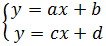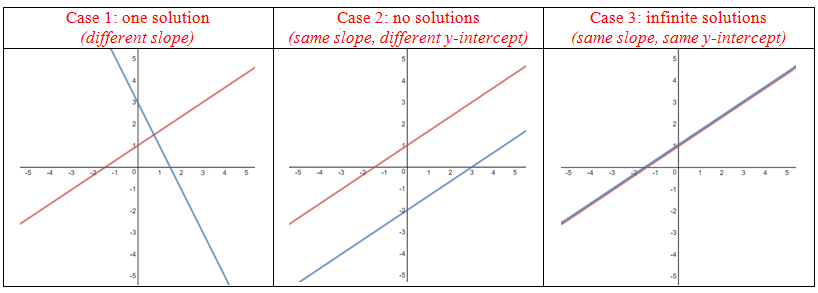# Determining number of solutions to linear equations

### Determining number of solutions to linear equations

Depending on whether and how the linear equations in a system touch each other, there will be different number of solutions to the system. There can be one solution, no solution and even infinite solution.

#### Lessons

• Introduction
$\bullet$ The solutions to a system of equations are the points of intersection of the graphs.
$\bullet$ For a system consisting of two linear equations:There are 3 cases to consider:• 1.
State whether each of the following systems have ONE, NONE, or INFINITE solutions

i) 3x + y = 7
4x + y = 7

ii) 6x + 2y = 10
3x + y = 5

iii) x - y = 3
3x - 3y = 6

• 2.
Find a value for c that will give the following system:
3y + 2cx = 6
y - 6x = 0

i) one solution

ii) no solutions